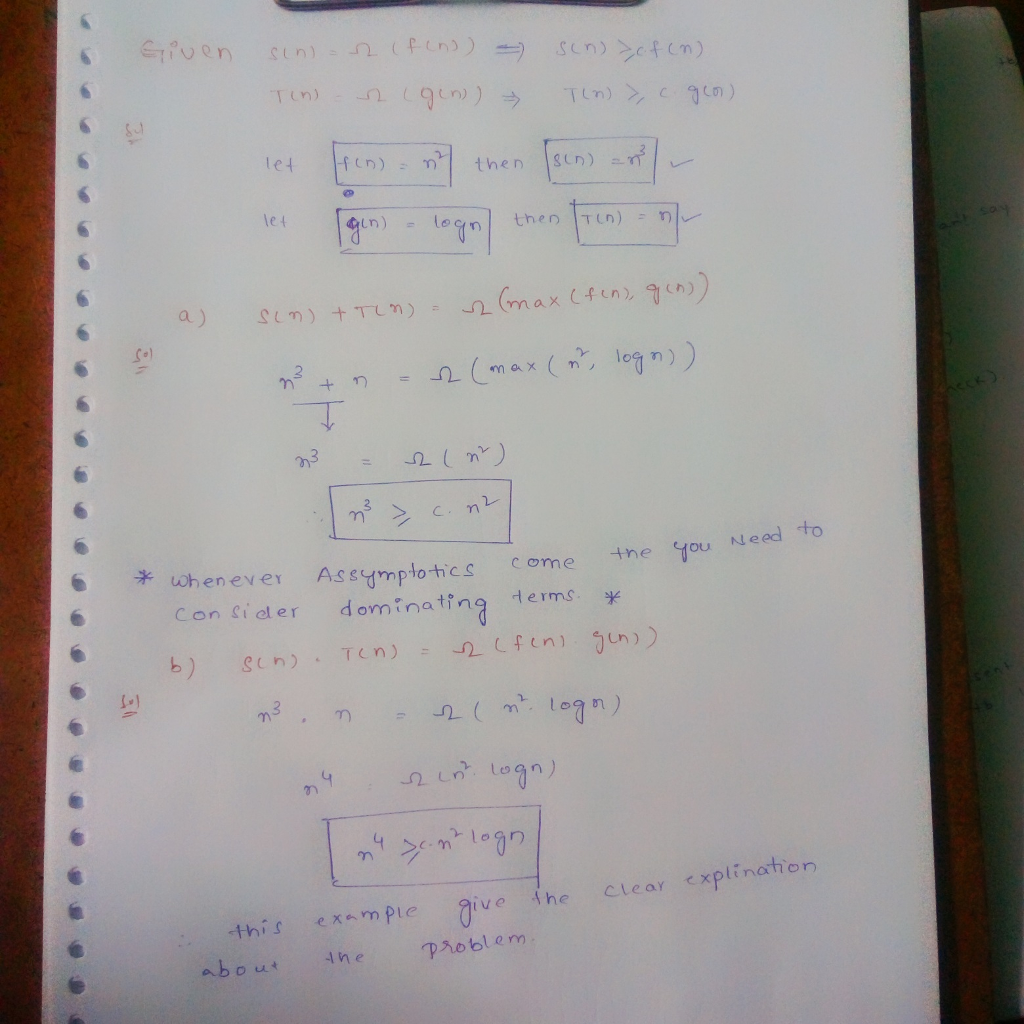# Homework Solution: Suppose S(n) = Ω(f(n)) and T(n) = Ω(g(n)), then which of the following statements are true? Ju…

Suppose S(n) = Ω(f(n)) and T(n) = Ω(g(n)), then which of the following statements are true? Justify your answer with reasoning. (Note: unless specified otherwise, all functions in this course are positive, non-decreasing, integer-valued functions. ) (a) S(n) + T(n) = Ω(max(f(n), g(n))). (b) S(n)T(n) = Ω(f(n)g(n)).